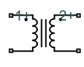# Mutual Inductor

Model two coupled inductors for circuit envelope analysis

• Library:
• RF Blockset / Circuit Envelope / Elements

•## Description

The Mutual Inductor block models an inductor within the RF Blockset™ circuit envelope simulation environment. For an introduction to RF simulation, see the example, Simulate High Frequency Components.

The block implements the relations

`$\begin{array}{c}{v}_{1}\left(t\right)={L}_{1}\frac{d}{dt}\left[{i}_{1}\left(t\right)\right]+M\frac{d}{dt}\left[{i}_{2}\left(t\right)\right]\\ {v}_{2}\left(t\right)=M\frac{d}{dt}\left[{i}_{1}\left(t\right)\right]+{L}_{2}\frac{d}{dt}\left[{i}_{2}\left(t\right)\right]\\ M=K\sqrt{{L}_{1}{L}_{2}}\end{array}$`

where:

• L1 and L2 represent inductances.

• M represents a mutual inductance with coefficient of coupling K.

• v1(t) and v2(t) represent the voltage across the terminals of the inductors at time t.

• i1(t) and i2(t) represent the current through the inductors at time t. The block uses standard dot notation to indicate the direction of positive current flow relative to a positive voltage.

RF Blockset current and voltage signals consist of in-phase (Ik) and quadrature (Qk) components at each frequency fk specified in the Configuration block:

`$\begin{array}{c}i\left(t\right)=\sum _{\left\{{f}_{k}\right\}}\left({i}_{{I}_{k}}\left(t\right)+j\cdot {i}_{{Q}_{k}}\left(t\right)\right){e}^{j\left(2\pi {f}_{k}\right)t}\\ v\left(t\right)=\sum _{\left\{{f}_{k}\right\}}\left({v}_{{I}_{k}}\left(t\right)+j\cdot {v}_{{Q}_{k}}\left(t\right)\right){e}^{j\left(2\pi {f}_{k}\right)t}\end{array}$`

## Parameters

expand all

Inductance of the first inductor, specified as a positive scalar in `H`, `nH`, `μH`, `mH`.

Inductance of the second inductor, specified as a positive scalar in `H`, `nH`, `μH`, `mH`.

Coefficient of coupling for the mutual inductance of the two inductors, specified as a scalar value from 0 through 1.

## Version History

Introduced in R2012a